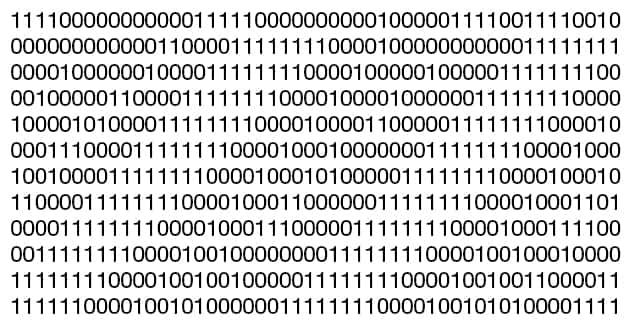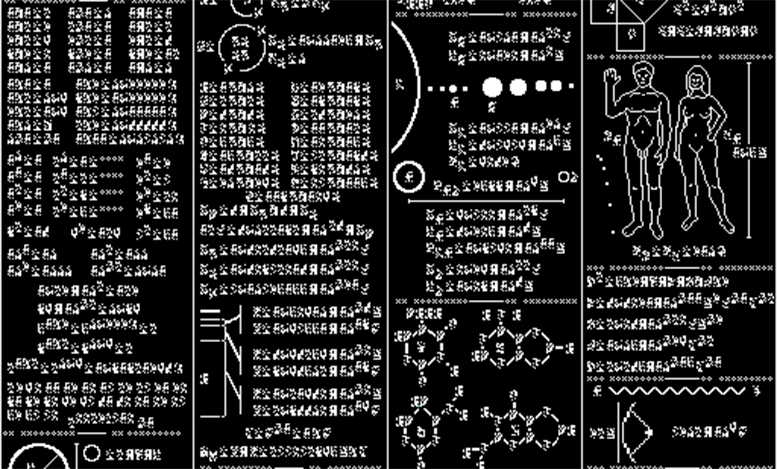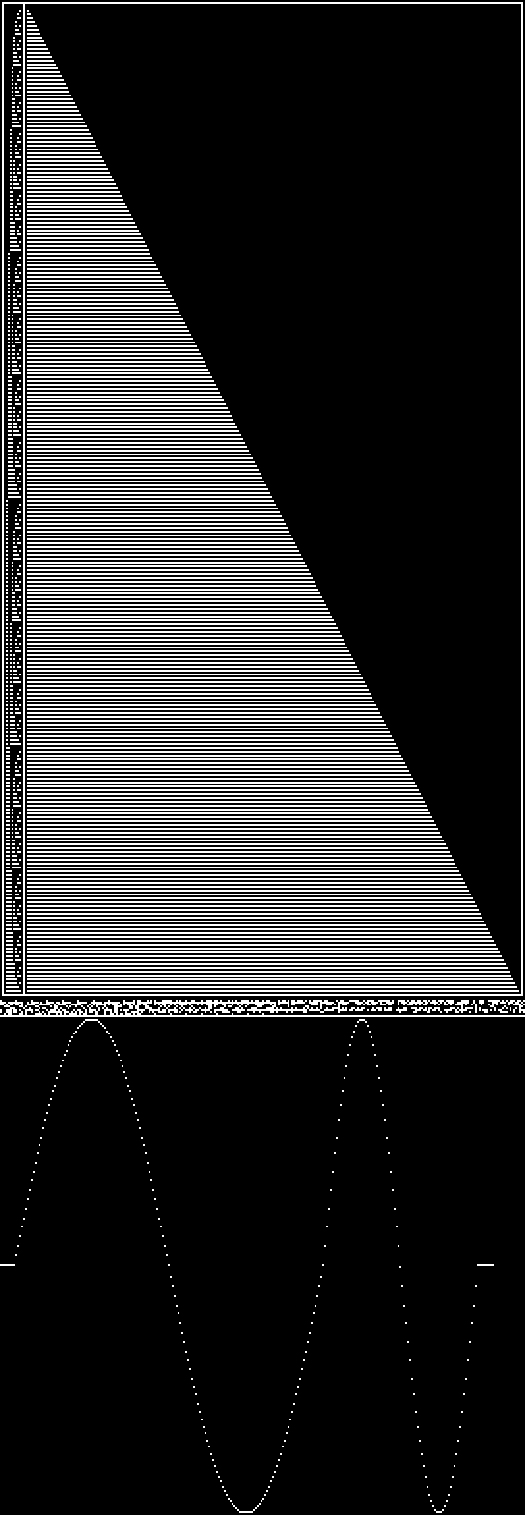# Tutoriales

### Tutorial - 1st transmision

Once it has been made clear to our extra-terrestrial listeners that we are sending them an intelligent signal, another complication arises: How can we explain things about ourselves without having to learn a whole new language? The answer lies in the so-called 'self-decodable messages', in which our colleagues at METI (Messaging Extra-terrestrial Intelligence) have played a key role.

These types of messages are based on the progressive introduction of information, similar to the process carried out in the 'Hello' section: in this section, we first introduced (implicitly) the concept of 'bit' and numbers, and then passed on to the concept of 'digital numbers in blocks of 8 bits'. The difficulty arises when we want to explain more complex concepts like, for example, music!

In order to make this possible we have defined a small 'dictionary' of symbols and concepts, each one described with an 8-bit block. The 'tutorial' section of our message presents the different concepts little by little, as if we were teaching a baby to talk. For example, a sequence of symbols meaning '0 + 0 = 0' is presented, followed by '0 + 1 = 1', then '1 + 0 = 1', '1 + 1 = 2', and so on, progressing to multiplications, divisions, square roots, the number Pi, trigonometric operations etc. As well as basic mathematical operations our tutorial also explains physical concepts such as electromagnetic waves, frequencies, the speed of light, and finally the concept of sound.

The last part of the tutorial section, necessary to interpret the digital music created by our artists, is a practical demonstration of simple sounds. Specifically, we use the well-known 8-bit blocks to encode a simple sine wave (i.e., a musical frequency) using exactly the same digital format as used by the different musical pieces. We start with a frequency of 100 hertz (for a tenth of a second), then 200 hertz, to 400, 800, and finally 1600 hertz. This implicitly shows the concept of harmonic frequencies. To end our tutorial, we combine several of these frequencies to demonstrate the concept of more complex sounds: 100 and 200 hertz at the same time; then 100, 200 and 400 at the same time etc., finishing with five combined frequencies (100, 200, 400, 800 and 1600 hertz). Our tutorial finishes with a brief silence, after which we are ready to start with the short musical pieces.message

### Tutorial - 2nd transmission

Once it has been made clear to our extra-terrestrial listeners that we are sending them an intelligent signal, another complication arises: How can we explain things about ourselves without having to learn a whole new language? The answer lies in the so-called 'self-decoding messages' (or ‘propaedeutic messages’), in which Dr. Yvan Dutil has a long expertise.

This kind of messages are based on the progressive introduction of information, similar to the process carried out in the 'Hello' section: therein, we first introduced (implicitly) the concept of 'bit', of numbers, and of prime numbers - everything in the same message. The difficulty arises when we want to explain more complex concepts like, for example, music!

In order to make this possible, Dr. Dutil defined, together with his colleague Stéphane Dumas (1970-2016), back in 1999 (later revised), a small ‘dictionary’ of symbols or concepts, each described with an image of a few pixels. The 'tutorial' section of our message presents the different concepts little by little, as if we were teaching a baby to talk. For example, the are symbols to represent numbers (from 0 to 9), others meaning physical units (like meter or second), others representing chemical elements, others which are math operators, physical concepts, or biological, astronomical… The message in itself starts by presenting basic numerical concepts like the binary coding of decimal numbers, or the basic mathematical operations: 1+1=2, 1-1=0, 1/1=1, etc. The message progressively evolves in complexity, going through more complex math operations, geometrical concepts, chemical elements, units of measure… up to the Solar system, the humans, or the DNA. Everything is contained in a small image of just 512 by 517 black and white pixels. This is actually the main difference between this tutorial and that created by METI experts which was sent with the first transmission in October 2017. In that occasion, we did without images to allow shrinking further the information and create a slightly more complete tutorial. The principle in both cases is the same: a message that progressively introduces the concepts, providing similar information on mathematics, physics, humanity and Cosmos. The METI tutorial does not require understanding of the message as an image, yet it may look like slightly more complex to be understood or decoded. The Dutil tutorial presents information graphically, which also allows better illustrating some of the concepts. By combining both approaches in different transmissions we increase the chances to be understood by our stellar neighbours.

The last part of our tutorial section, created by IEEC experts and necessary to interpret the digital music created by our artists, is an illustration and practical demonstration of the concept of digital music and simple sounds. First, we graphically explain the concept of ‘byte’ (8 bits) by showing a binary count from 0 to 255 and illustrating it with the adequate number of white pixels on a black background. Afterwards, we generate a brief sequence of bytes which codes a simple sine wave (with two musical frequencies) illustrated with a small image. After this, the tutorial leaves the graphical illustrations to enter the purely digital domain: we repeat the binary count from 0 to 255 with a simple sequence of bytes, and finally we transmit a small example of digital music using exactly the same format than the several music tracks from Sónar artists. Specifically, we start with a frequency of 100 hertz (for a tenth of a second), then 200 hertz, 400, 800, and finally 1600 hertz. This implicitly shows the concept of harmonic frequencies. To end our tutorial, we combine several of these frequencies to demonstrate the concept of more complex sounds: 100 and 200 hertz at the same time; then 100, 200 and 400 at the same time etc., finishing with five combined frequencies (100, 200, 400, 800 and 1600 hertz). Our tutorial finishes with a brief silence, after which we are ready to start with the short musical pieces.